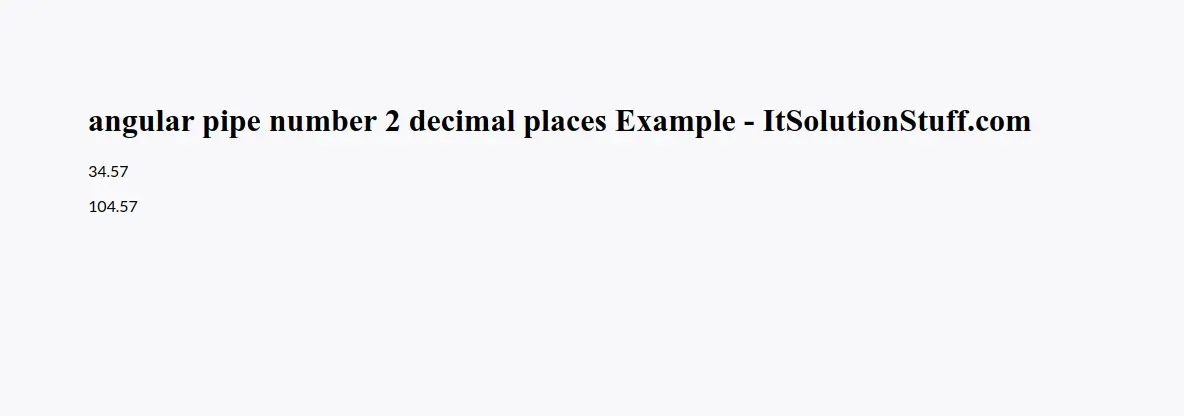# Angular Pipe Number 2 Decimal Places Example

By Hardik Savani October 20, 2023 Category : Angular

Today our leading topic is angular pipe number 2 decimal places. Here you will learn angular pipe decimal 2 digits. you'll learn angular decimal pipe 2 digits. if you want to see example of angular 2 digit after decimal point pipe then you are a right place.

you can easily use pipe for 2 digits after decimal point in angular 7, angular 8, angular 9, angular 10, angular 11, angular 12, angular 13, angular 14, angular 15 and angular 16 version.

here, you have to follow few step to create simple example of pipe 2 digits after decimal point.

In this simple example, you can see i take two variable and it's has 3 or 4 digits after decimal point. so let's see how to display with 2 digits after decimal point.

app/app.component.ts

`import { Component, VERSION } from "@angular/core";  @Component({  selector: "my-app",  templateUrl: "./app.component.html",  styleUrls: ["./app.component.css"]})export class AppComponent {  name = "Angular " + VERSION.major;    myNumber = 34.5673;  myNumber2 = 104.566445;}`

Ok, now we can use custom pipe in html file, so let's write it.

app/app.component.html

`<h1>angular pipe number 2 decimal places Example - ItSolutionStuff.com</h1>  <p>{{ myNumber | number : '1.2-2' }}</p><p>{{ myNumber2 | number : '1.2-2' }}</p>`

Output: